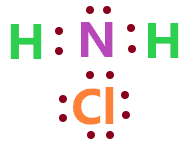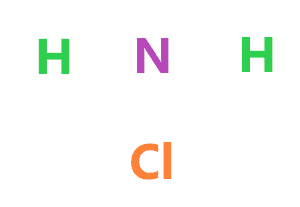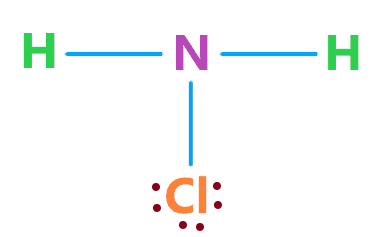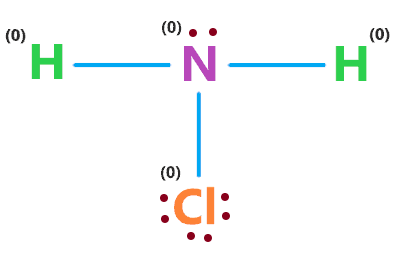# NH2Cl lewis dot structure, molecular geometry, polar or nonpolar, hybridization

Home  > Chemistry Article > NH2Cl lewis structure and its molecular geometryNH2Cl is simply a chloramine that is also called Monochloramine, it appears as a colorless gas to yellow liquid with a strong pungent odor, long exposure to this can cause irritation in the eyes and rashes on the skin.

In this article, we will discuss NH2Cl lewis structure, molecular geometry, polar or nonpolar, its hybridization, etc.

Monochloroamine is one of three chloramines of ammonia, the other two are nitrogen trichloride and dichloramine.

 Name of Molecule Chloramine Chemical formula NH2Cl Molecular geometry of NH2Cl Trigonal pyramidal Electron geometry of NH2Cl Tetrahedral Hybridization Sp3 Nature Polar molecule Total Valence electron for NH2Cl 14
Page Contents

## How to draw lewis structure of NH2Cl?

NH2Cl lewis structure contains two N-H bonds and one N-Cl bond, the nitrogen atom in the central position and all other atoms are in the surrounding position. There is one lone pair present on the central atom of the NH2Cl lewis structure.

The drawing of NH2Cl lewis’s structure is simple and easy to understand, Just follow the steps we have written for you.

## Follow some steps for drawing the lewis dot structure for NH2Cl

1. Count total valence electron in NH2Cl

Valence electrons are found in the outer shell of the atom that can form a chemical bond with another atom. To get the total valence electron in NH2Cl, look at the group number of individual atoms – nitrogen, hydrogen, and chlorine.

The nitrogen atom belongs to the pnictogens family and has a group number 15, hence, its valence electron is 5, the chlorine atom belongs to the halogen family and is situated in group number 17, hence, its valence electron is 7.

The hydrogen atom is situated in the first row of the Periodic Table and has period group number 1, hence, its valence electron is 1.

⇒ Total number of the valence electrons in nitrogen = 5

⇒ Total number of the valence electrons in hydrogen = 1

⇒ Total number of the valence electrons in chlorine = 7

∴ Total number of valence electrons available for the NH2Cl Lewis structure = 5 + 1(2) + 7 = 14 valence electrons         [∴ NH2Cl molecule has 1 nitrogen, 2 hydrogen and 1 chlorine atom]2. Find the least electronegative atom and place it at center

The more electropositive element is preferred for holding the position of the central region in the lewis diagram. Let’s take a look, which should be the central atom for the NH2Cl lewis structure.

The hydrogen can never be the central atom since it can form a maximum of one covalent bond. So, among nitrogen and chlorine, look at their electronegativity value.

The electronegativity of nitrogen is 3.04 and for chlorine, it is 3.16. Clearly, the nitrogen atom is less electronegative or more electropositive, hence, put the nitrogen atom in the central position, the hydrogen atom on either side of it.

And chlorine atom should either be above or below the nitrogen atom as you want.3. Connect outer atoms to central atom with a single bond

Draw the skeletal structure of NH2Cl by connecting each outer atom to the central atom with a single bond.Look at the above structure and determine the number of valence electrons is used till now.

In the above structure, three single bonds are used and every single bond contains  2 electrons, hence, (3 × 2) = 6 valence electrons are used from 14 total available valence electrons for drawing the lewis structure of NH2Cl.

∴ (14 – 6) = 8 valence electrons

So, we are left with 8 valence electrons more.

4. Place remaining electrons on outer atoms and complete their octet

In this step, we have to complete the octet of more electronegative atoms means the atom is present in the surrounding position(other than the central atom).

In the case of NH2Cl, the hydrogen and chlorine atoms are the outer atoms, hence, we need to complete their octet first.

A hydrogen atom needs only 2 electrons in its valence shell to complete the octet whereas the chlorine atom needs 8 electrons in its valence shell to complete the octet.

As we have 8 remaining valence electrons, therefore, put these electrons on outer atoms till they complete their octet.In the above structure, the chlorine atom has 8 electrons(6 represented as dots + 2 electrons in a single bond), hence, it completed its octet.

The hydrogen atoms already completed their octet since they have 2 electrons(one single bond) in their valence shell.

Now again count the valence electrons are used till now. In the above structure,  (Three single bonds mean 6 electrons + 6 electrons represented as dots) = 12 valence electrons are used till now from 14 total of available valence electrons for NH2Cl lewis structure.

Therefore, we are left with only 2 more valence electrons.

5. Complete the octet of the central atom

In the NH2Cl molecule, nitrogen is the central atom and it needs 8 electrons in its valence shell to fulfill the octet.

By looking at the 4th step structure, we see that the nitrogen atom is attached with three single bonds means it has already 6 electrons in its valence shell, hence, it needs only two more valence electrons to complete the octet.

We already have 2 remaining valence electrons, hence, just put these 2 valence electrons over the nitrogen atom and fulfill its octet.### NH2Cl lewis structure

That’s all, all the atoms in the above NH2Cl lewis structure fulfilled their octet and attains stability and we also used all the total valence electrons that are available for drawing it.

Now we will check the above structure stability by evaluating the formal charge for each atom.

6. Check the stability with the help of a formal charge concept

To calculate the formal charge on an atom. Use the formula given below-

⇒ Formal charge = (valence electrons – nonbonding electrons –  1/2 bonding electrons)

The nonbonding electrons are basically lone pair electrons and bonding electrons are shared electrons that are found in between the atoms.

Let’s calculate the formal charge for nitrogen, chlorine, and hydrogen atoms in the NH2Cl lewis structure.

For nitrogen atom:

⇒ Valence electrons of nitrogen = 5

⇒ Nonbonding electrons on nitrogen= 2

⇒ Bonding electrons around nitrogen(3 single bond) = 6

∴ (5 – 2 – 6/2) = 0 formal charge on nitrogen central atom.

For hydrogen atom

⇒ Valence electrons of hydrogen = 1

⇒ Nonbonding electrons on hydrogen = 0

⇒ Bonding electrons around hydrogen (1 single bond) = 2

∴ (1 – 0 – 2/2) = 0 formal charge on the hydrogen atom.

For chlorine atom

⇒ Valence electrons of chlorine = 7

⇒ Nonbonding electrons on chlorine =6

⇒ Bonding electrons around chlorine (1 single bond) = 2

∴ (7 – 6 – 2/2) = 0 formal charge on the chlorine atom.So, all-atom in the above structure gets a formal charge equal to zero, hence, this is our most stable and appropriate lewis structure of NH2Cl.

## What is the molecular geometry of NH2Cl?

The molecular geometry of NH2Cl is a Trigonal pyramid because the central atom nitrogen is connected to three atoms(two hydrogens and one chlorine), and there is one lone pair present on the nitrogen central atom, hence, it is an AX3N type molecule.

A = central atom in lewis diagram

X = Bonded atoms to the central atom

N = Number of lone pair on central atom

The AX3N type shows that the NH2Cl molecule has four regions of electron density(3 bond pairs + 1 lone pair), hence, according to the VSEPR chart, its molecular geometry will be trigonal pyramidal and its electron geometry will be tetrahedral.## Hybridization of NH2Cl

Since the central atom of NH2Cl is surrounded by four regions of electron density(3 bonded atoms + 1 lone pair), its steric number will be 4.

For a steric number of 4, the hybridization will be Sp3 means 25% s-character and 75% p-character.

Therefore, the hybridization of NH2Cl is Sp3.

## Is NH2Cl polar or nonpolar?

Is NH2Cl polar or non-polar? NH2Cl is a polar molecule because of its asymmetrical shape i.e. trigonal pyramidal that ensures that vectors will not cancel out in this molecule which results in some net dipole moment that makes NH2Cl polar in nature.

Basically, NH2Cl forms three types of bonds – two N-H and one N-Cl.

⇒ The electronegativity of hydrogen = 2.2

⇒ The electronegativity of nitrogen = 3.04

⇒ The electronegativity of chlorine = 3.16

∴ The difference of electronegativity in N-H bond = 0.84

Hence, the N-H bonds in the NH2Cl molecule are polar in nature since their electronegativity difference is more than 0.5.

The “N-Cl” bond also is polar because chlorine is more electronegative than nitrogen.

Therefore, the charges distributed unequally or asymmetrically over the atoms – hydrogen, chlorine, and nitrogen in the NH2Cl molecule that results in some net dipole moment and due to the trigonal pyramidal shape of NH2Cl these net dipole moments retain permanently in the molecule.

All these make, NH2Cl polar in nature.

## FAQ

### The total number of lone pairs present in the lewis structure of NH2Cl?

The lone pair are represented as dots in the lewis diagram. According to the NH2Cl lewis structure, there is a total of 8 dots present(2 dots on the nitrogen atom and 6 dots around the chlorine atom).

Hence, the number of lone pairs in the NH2Cl lewis structure is 4

### The total number of bond pairs present in the lewis structure of NH2Cl?

The bond pairs simply are the bonding electrons that are found between the atoms, generally in the covalent bond.

Two bonding electron between the atoms forms a single covalent bond.

Four bonding electron between the atoms forms a double covalent bond.

Now, as per NH2Cl lewis structure, the central atom nitrogen is attached with three single covalent bonds, and one single covalent bond means 2 bonding electrons.

Hence, total bonding electrons is (3 × 2) = 6 bonding electrons that make 3 bond pairs.

Properties of Chloramine

• Its appearance varies from colorless to yellow liquid and has a very strong pungent odor.
• It has a melting point of −66 °C and its boiling point is unknown because “it is too unstable to have its boiling point measured”.
• The common use of chloramine is as a disinfectant for water, also, in swimming pool disinfection.
• At temperatures above −40 °C, the pure chloramine decomposes violently.
• It is soluble in water and ether, but less soluble in CHCl3 and CCl4.
• At low concentrations, the Chloramine is thermally slightly more stable.
• The long-term inhalation of Chloramine gas can cause nausea and vomiting.

Preparation and reactions of Chloramine

Chloramine is prepared in three forms, in dilute aqueous solution, in gaseous form, and in pure form.

Pure chloramine is prepared by reacting 2 moles of fluoroamine with calcium chloride.

⇒ 2 NH2F + CaCl2 → 2 NH2Cl + CaF2

Chloramine in gas form can be prepared by the reaction of 2 moles of gaseous ammonia with simple chlorine gas.

⇒ 2NH3 + Cl2 ⇌ NH2Cl + NH4Cl

The diluted solution of NH2Cl is prepared by the simple reaction of one mole of ammonia with one mole of sodium hypochlorite.

⇒ NH3 + NaOCl → NH2Cl + NaOH

## Summary

• The total valence electron is available for drawing the NH2Cl Lewis structure is 14.
• The molecular geometry of NH2Cl is trigonal pyramidal and its electron geometry is tetrahedral.
• The lewis structure of NH2Cl has 6 bonding electrons and 8 nonbonding electrons.
• The hybridization of NH2Cl is Sp3.
• NH2Cl is polar because of its asymmetrical shapes that cause unequal charge distribution, resulting in some net dipole moment.
##### Subscribe to Blog via Email

Join 2 other subscribers

Share it...